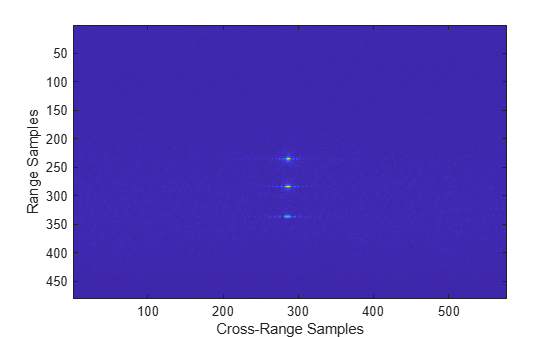# rangeMigrationLFM

Range migration image formation algorithm for linear FM waveform

## Syntax

``slcimg = rangeMigrationLFM(raw,waveform,fc,v,rc)``
``slcimg = rangeMigrationLFM(raw,waveform,fc,v,rc,Name=Value)``

## Description

example

````slcimg = rangeMigrationLFM(raw,waveform,fc,v,rc)` returns a single-look complex image of raw synthetic aperture radar (SAR) data obtained with a linear frequency modulated (LFM) pulse waveform. The function uses the range migration algorithm.```
````slcimg = rangeMigrationLFM(raw,waveform,fc,v,rc,Name=Value)` specifies additional inputs using name-value arguments. You can specify the squint angle of the antenna and the signal propagation speed.```

## Examples

collapse all

Load a file that contains simulated unfocused synthetic aperture radar (SAR) data obtained with a linear frequency modulated waveform. The waveform has a sample rate of 45 MHz, a pulse width of 3 microseconds, a pulse repetition frequency of 960 seconds, and a sweep bandwidth of c/10 Hz, where c is the numerical value of the speed of light.

```data = load("RangeMigrationLFMExampleData"); raw = data.raw; wvf = phased.LinearFMWaveform(SampleRate=45e6, ... PulseWidth=3e-6,PRF=960,SweepBandwidth=physconst("LightSpeed")/10);```

The system works at an operating frequency of 4 GHz. The platform moves at a velocity of 100 meters per second and the distance between the beam center on the ground and the radar is 1118 meters.

```fc = 4e9; v = 100; rc = 1118;```

Generate a single-look complex image from the data. Plot the image.

```slcimg = rangeMigrationLFM(raw,wvf,fc,v,rc); imagesc(abs(slcimg)) xlabel("Cross-Range Samples") ylabel("Range Samples")```## Input Arguments

collapse all

Raw SAR data, specified as a matrix. The data is the unfocused in-phase and quadrature (I/Q) raw data collected by the SAR system. The rows of `raw` correspond to the along-range samples. The columns of `raw` correspond to the pulses received as the platform moves along the cross-range direction. `raw` must have at least two rows.

Data Types: `double`

Input waveform, specified as a `phased.LinearFMWaveform` object.

Example: `phased.LinearFMWaveform(SampleRate=500e3,'SweepBandwidth',200e3,PulseWidth=1e-3,PRF=1e3)`

Data Types: `double`

Operating frequency, specified as a positive scalar. Units are in Hz.

Example: `2.8e9` Hz specifies a typical S-band operating-frequency value for airport and weather radar systems.

Data Types: `double`

Platform velocity, specified as a positive scalar. Units are in meters per second.

Data Types: `double`

Distance between radar and beam center on the ground, specified as a positive scalar. Units are in meters.

Data Types: `double`

### Name-Value Arguments

Specify optional pairs of arguments as `Name1=Value1,...,NameN=ValueN`, where `Name` is the argument name and `Value` is the corresponding value. Name-value arguments must appear after other arguments, but the order of the pairs does not matter.

Example: `SquintAngle=45,PropagationSpeed=343`

Squint angle of the antenna from the broadside direction in degrees, specified as a scalar in the range `(-90, 90)`.

Data Types: `double`

Signal propagation speed in meters per second, specified as a positive scalar.

Example: `343` meters per second approximates the speed of sound at sea level and at a temperature of 20 °C under normal atmospheric conditions.

Data Types: `double`

## Output Arguments

collapse all

Single-look complex (SLC) image, returned as a matrix. `slcimg` is the same size as `raw` and contains the focused data processed by the range migration algorithm.

## Version History

Introduced in R2022a